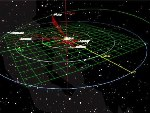# How to calculate the synchronous orbit?

## Synchronous orbitAutomatic translation Category: probes and satellites
Updated June 22, 2014
The synchronous orbit is the orbit that allows a satellite to make a revolution around the planet while the planet makes one tour around itself. This means that if the orbit has an inclination and eccentricity equal to 0, then the satellite will appear from the ground of the planet, "immobile", still hanging in the sky at the same position above the equator.
When the inclination of the plane of the satellite orbit is equatorial (inclination ≠ 0), the satellite appears to oscillate from north to south, above the equator of the planet.
When the satellite's orbit is elliptical (eccentricity ≠ 0), the satellite appears to oscillate from East to West.
When the inclination of the satellite orbit and the eccentricity are both different from 0, then the satellite moves across the sky producing a figure 8-shaped, called analemma.
It does not exist in the solar system natural satellite, in synchronous orbit planet. On Earth, almost circular orbits are used by synchronous artificial communication satellites, these are the famous geostationary orbits.
A satellite in a synchronous orbit is always in synchronous rotation (see note) because the satellite is blocked by tidal forces.
The geostationary orbit is a geosynchronous orbit, which has an inclination and a zero eccentricity. Above the geostationary orbit at 35,796 km altitude above the equator is a belt 230 km called "graveyard orbit" or "orbit trash" is the cemetery of satellites end of life.

Nota: be careful not to confuse the synchronous orbit of a satellite with a satellite synchronous rotation. The synchronous orbit is the orbit that allows a satellite to take a tour around the planet while the planet makes one revolution around itself. In the rotation synchronous satellite puts the same time to rotate about its axis for rotation about the planet. In the case of synchronous orbit, the satellite is always in the same place in the sky and in the case of synchronous rotation, the satellite always presents the same face to the planet. This is the case of the moon having a rotational period equal to the period of revolution (27.3217 days), it always presents the same face in the Earth but it moves in the air since it is not located on a synchronous orbit.

## Where is the synchronous orbits of the planets?

How to calculate the synchronous orbits of the planets?

The synchronous orbit planets is calculated using the law of universal gravitation which boils down to: h=√3(G*M*T2/4π2)-R

h = Height of the artificial satellite
G = Constant of gravitation (6.67*10-11)
M = Mass of the planet
T = Rotational period of the planet
R = Radius of the planet
The geosynchronous orbit (synchronous orbit of the Earth) is at an altitude of 35,796 km (≈ 36,000 km) and has a semi-major axis of 42,167 km.
Excel formula used in this table to calculate the altitude of the satellite in synchronous orbit of the planet:
=((((G*M*T^2)/(4*PI()^2))^(1/3))-R*1000)/1000
Excel formula used to calculate the semi-major axis of the synchronous orbit of the planet:
=((((G*M*T^2)/(4*PI()^2))^(1/3)))/1000

 Planets Mass(1024 kg) Volume(1012 km3) Radius(km) Rotationperiod(s) Synchronousorbit altitude(km) Synchronous orbitSemi-major axis(km) Satellitespeed(km/s) Mercury 0.3302 0.06083 2439.74 5 053 137 240 025 242 464 0.30 Venus 4.8685 0.92843 6051.83 20 939 611 1 527 832 1 533 883 0.46 Earth 5.9736 1.08321 6371.01 86 163 35 796 42 167 3.07 Mars 0.64185 0.16318 3389.95 88 400 17 002 20 392 1.45 Jupiter 1898.6 1431.28 69910.97 35 629 89 811 159 722 28.17 Saturn 568.46 827.13 58232.00 38 256 53 811 112 043 18.40 Uranus 86.81 68.33 25361.46 61 894 57 173 82 534 8.38 Neptune 102.43 62.526 24622.04 57 837 58 739 83 361 9.06 Europa 0.048 0.01593 1560.90 306 822 18 134 19 694 0.40

### Table: synchronous orbits of the planets and moons of planets.

 1997 © Astronoo.com − Astronomy, Astrophysics, Evolution and Earth science. Contact    Mentions légalesWhat is an apsis?The satellites measure
the underwater relief...Explanation of "8"
of the analemma...Rosetta and Philae...With or without horizon,
where is the space station?